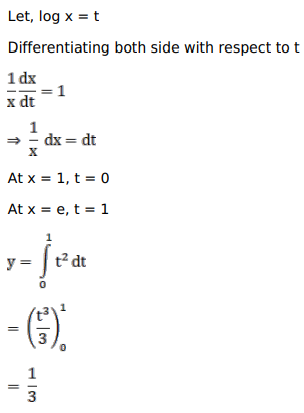# Mark against the correct answer in the following:

Question:

Mark $(\sqrt{ })$ against the correct answer in the following:

$\int_{1}^{e} \frac{(\log x)^{2}}{x} d x=?$

A. $\frac{1}{3}$

B. $\frac{1}{3} \mathrm{e}^{3}$

C. $\frac{1}{3}\left(\mathrm{e}^{3}-1\right)$

D. none of these

Solution: